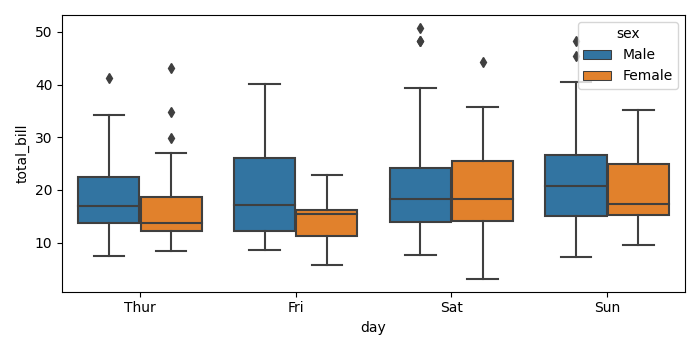# How to make a grouped boxplot graph in matplotlib?

To make a grouped boxplot graph in matplotlib, we can take the following steps −

• Import matplotlib.pyplot and seaborn.
• Set the figure size and adjust the padding between and around the subplots.
• Load an example Seaborn dataset from the online repository.
• Make a boxplot with male and female group in a single day.
• To display the figure, use show() method.

## Example

import seaborn as sns
import matplotlib.pyplot as plt

# Set the figure size
plt.rcParams["figure.figsize"] = [7.00, 3.50]
plt.rcParams["figure.autolayout"] = True

# Import a Seaborn dataset

# Create a grouped boxplot
sns.boxplot(x=data['day'], y=data['total_bill'], hue=data['sex'])

plt.show()

## Output

It will produce the following outputUpdated on: 19-Oct-2021

2K+ Views